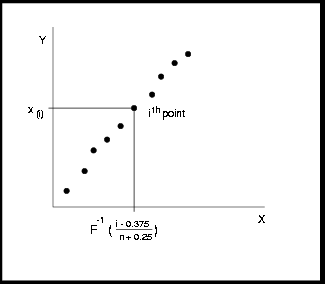# The UNIVARIATE Procedure

### Construction of Quantile-Quantile and Probability Plots

Figure 4.14 illustrates how a Q-Q plot is constructed for a specified theoretical distribution. First, the n nonmissing values of the variable are ordered from smallest to largest:

Then the ith ordered value is plotted as a point whose y-coordinate is and whose x-coordinate is , where is the specified distribution with zero location parameter and unit scale parameter.

You can modify the adjustment constants –0.375 and 0.25 with the RANKADJ= and NADJ= options. This default combination is recommended by Blom (1958). For additional information, see Chambers et al. (1983). Because is a quantile of the empirical cumulative distribution function (ECDF), a Q-Q plot compares quantiles of the ECDF with quantiles of a theoretical distribution. Probability plots (see the section PROBPLOT Statement) are constructed the same way, except that the x-axis is scaled nonlinearly in percentiles.

Figure 4.14: Construction of a Q-Q Plot